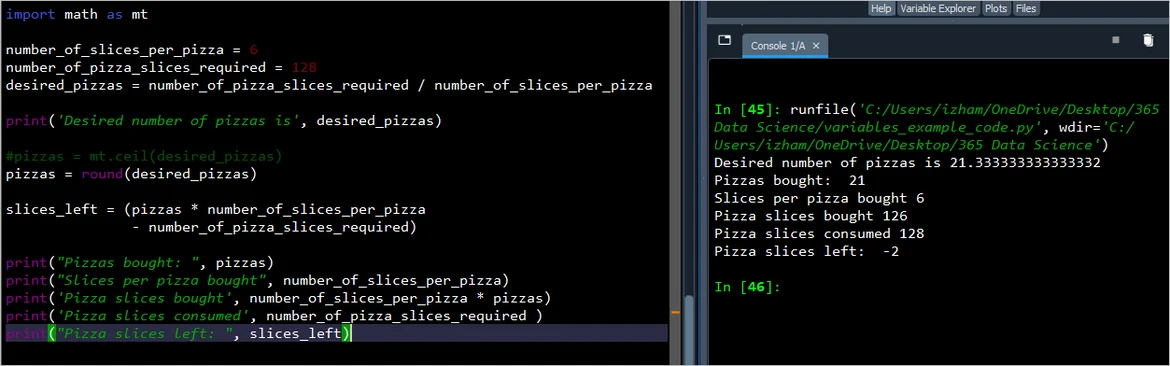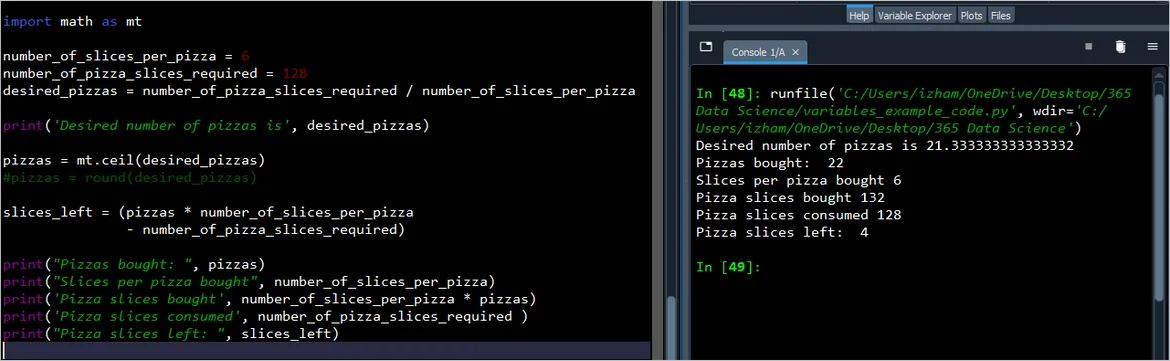19 Dec 2021

Posted on:

28 Oct 2021

2

# Resolved:floor division to determine the minimum number of pizzas

Is the addition of 5 to total_slices when defining number_of_pizzas arbitrary so that a floor division will be required? any number from 3 to 7 could have been used?

Posted on:

07 Nov 2021

3

OR it'd be more easier if we'd do it like this
total_slices//6 + 1
because all we need is one extra pizza everytime so thats one more way to do it

Posted on:

07 Nov 2021

0

But wait, where that 1 come from?

if they eat 16, and 3 pizzas are 18, and the floow division is 2 the 1 is the aditional slice?

But the professor´s example really lost me because I didn´t get the idea

Posted on:

07 Nov 2021

1

1 is actually added for that one extra pizza because floor division would always return lowest value
so when you do 16//6 you would get 2 but 2 pizzas would only make up for 12 slices so you need 3 pizzas thats why I added a extra 1 by myself coz everytime you're going to need 1 extra pizza to make up for total slices needed

Posted on:

08 Nov 2021

3

Hey Luke,

Thank you for the question!

The pizza shop only offers pizzas that have 6 slices. Therefore, the possible number of slices that one could get is 6, 12, 18, 24, 30, ... Imagine that we do not know a priori what the value of `total_slices` is. Whichever the value, we want to make sure that everybody gets a piece.

Let's change the task a little and say that we have only two friends - Bob and Ann. Bob will eat 4 slices, Ann will eat 3 slices and so `total_slices = 7`. We want to figure out the number of pizzas we need. 7//6 = 1, that won't be enough. 8//6 = 1 -> that won't be enough either. The number that will ensure we obtain 2 is 5 -> (7+5)//6 = 2. The nice thing about adding 5 is that, regardless of the value of `total_slices`, it will always help you get the correct amounts of pizzas.

In the example above, one can argue that all numbers from 5 through 10 would have done the job. That is true unless we change the number of total slices from 7 to 11. We would still need two pizzas. However, (11+10)//6 = 3, whereas (11+5)//6 = 2.

Hope this helps!

Kind regards,
365 Hristina

Posted on:

08 Nov 2021

1

Yes, I understand now. In the instance where two people eat just one slice into an additional pizza, the addition of 5 extra slices ensures the next whole number of pizzas. Thank you all!

Posted on:

10 Nov 2021

0

Adding the 5 is only to get a floor number?

If it so, we are taking the number arbitrarily to get a floor number, but, how could we get that numbers to form an operation and get a floor number in future for other cases, and the last example, you took 10 instead of 5, is the solution still right?

Posted on:

10 Nov 2021

0

``````# Bob, Ann, Jane and Ashwin want to order pizza - They know they will each eat
# 4 slices of pizza. The local pizza shop sells pizza of only 6 slices
# what is the minimun number of pizza needed - Use floor division

Bob = 4 #slices
Ann = 4 #slices
Jane = 4 #slices
Ashwin = 4 #slices

Total_Slices = 4*4 #total slices needed
Shop_pizza = 6 #slices only

pizza_needed = Total_Slices // Shop_pizza + 1 # Floor division gives us 2
#But 2 pizzas are only 12 slices, and it is not enough to eat
print('They need to buy',pizza_needed, 'pizzas to eat the group of friends')

print('\n----------------')

Remaining_slices = Shop_pizza * 3 - Total_Slices
print('And still remaining', Remaining_slices, 'Slices available for later on road')
``````
Posted on:

10 Nov 2021

1

Hey Arnaldo,

Here is an example of how the problem can be generalized to work for any number of slices per pizza as well as any number of total slices:

``````slices_per_pizza = int(input('Please, enter the slices per pizza:>>>'))
total_slices = int(input('Please, enter a total number of slices:>>> '))

pizzas = (total_slices + slices_per_pizza - 1)//slices_per_pizza

print(f'The number of pizzas needed is {pizzas}.')
``````

Try it out for different values of the variables. In Giles' example, `slices_per_pizza = 6` and `total_slices = 16`.

Kind regards,
365 Hristina

Posted on:

02 Dec 2021

1

Any reason why we don't round up the resulting value?

``````number_of_slices_per_pizza = 6
number_of_pizza_slices_required = 4 * 4
desired_pizzas = number_of_pizza_slices_required/number_of_slices_per_pizza
pizzas = round(desired_pizzas)
slices_left = pizzas * 6 - number_of_pizza_slices_required
print("Pizzas bought: ", pizzas)
print("Pizza slices left: ", slices_left)
``````
Posted on:

19 Dec 2021

0

I had a similar flow of thought to yours Faith.

I noticed that when we use the default "round" function it basically rounds to the closest integer (with values less than or equal to the exact midpoint between the two integers rounding down; e.g.: 20.5 rounds to 20, 20.3 rounds to 20, and 20.51 rounds to 21)

Using round can be slightly problematic here if we allow the number of slices required to vary.
For example, if we needed 128 slices and the pizzas each had 6 slices, we would need approx. 21.33 pizzas.
However, round would estimate this to be 21 – whereas we still need an extra .33 pizzas – so ideally we want 22 pizzas.

# Using round estimates the required 21.33 pizzas to 21, essentially resulting in a shortfall.# Importing the in-built "math" module and using its "ceil" method estimates the required 21.33 pizzas to 22 as required.APPENDIX (code used with second screenshot scenario; to review first scenario swap commenting in section where "pizzas" variable is defined):

import math as mt

number_of_slices_per_pizza = 6
number_of_pizza_slices_required = 128
desired_pizzas = number_of_pizza_slices_required / number_of_slices_per_pizza

print('Desired number of pizzas is', desired_pizzas)

pizzas = mt.ceil(desired_pizzas)
#pizzas = round(desired_pizzas)

slices_left = (pizzas * number_of_slices_per_pizza
- number_of_pizza_slices_required)

print("Pizzas bought: ", pizzas)
print("Slices per pizza bought", number_of_slices_per_pizza)
print('Pizza slices bought', number_of_slices_per_pizza * pizzas)
print('Pizza slices consumed', number_of_pizza_slices_required )
print("Pizza slices left: ", slices_left)